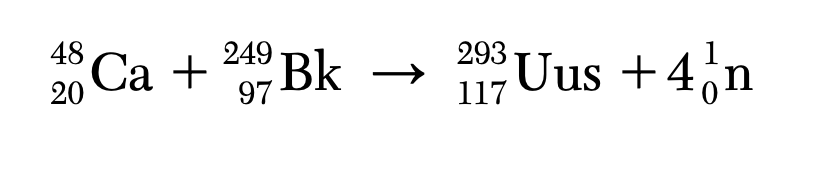top of page
Search

# Element 117, Uus, has been synthesized and has at least two isotopes, Uus-293 and Uus-294. Atoms of

Element 117, Uus, has been synthesized and has at least two isotopes, Uus-293 and Uus-294. Atoms of Uus-293 can be made by bombarding Bk-249 with Ca-48 in a reaction represented by the nuclear equation below.The Bk-249 has a half-life of 320. days, decays by beta emission, and also emits gamma rays. 83 Determine the fraction of Bk-249 that remains unchanged after 960. days. 

Explanation: We are told that the half life of Bk-249 is 320 days. A half life is the amount of time that is needed for an isotope to decay to one half of the original amount. 960 days/ 320 days = 3 half lives. Each arrow below represents a half life.

1----> 1/2 ---> 1/4 ---> 1/8

84 State, in terms of both protons and neutrons, why Uus-293 and Uus-294 are isotopes of the same element. 

Explanation: Isotopes of the same element have the same number of protons but different number of neutrons.

Answer: Uus-293 and Uus-294 are isotopes of the same element because they have the same number of protons but different number of electrons.

85 Complete the nuclear equation in your answer booklet for the alpha decay of Uus-294 by writing a notation for the missing product.Explanation: In order to balance a nuclear equation, the sum of top numbers (masses) must be equal on the left and right sides. Similarly, bottom numbers (atomic numbers) must be equal as well. So let's find what is missing.

TOP: 294 = 4+x

x= 290

Bottom: 117 = 2+y

y=115

Now we can check which element has an atomic number of 115 on the Periodic Table. It turns out to be Uup